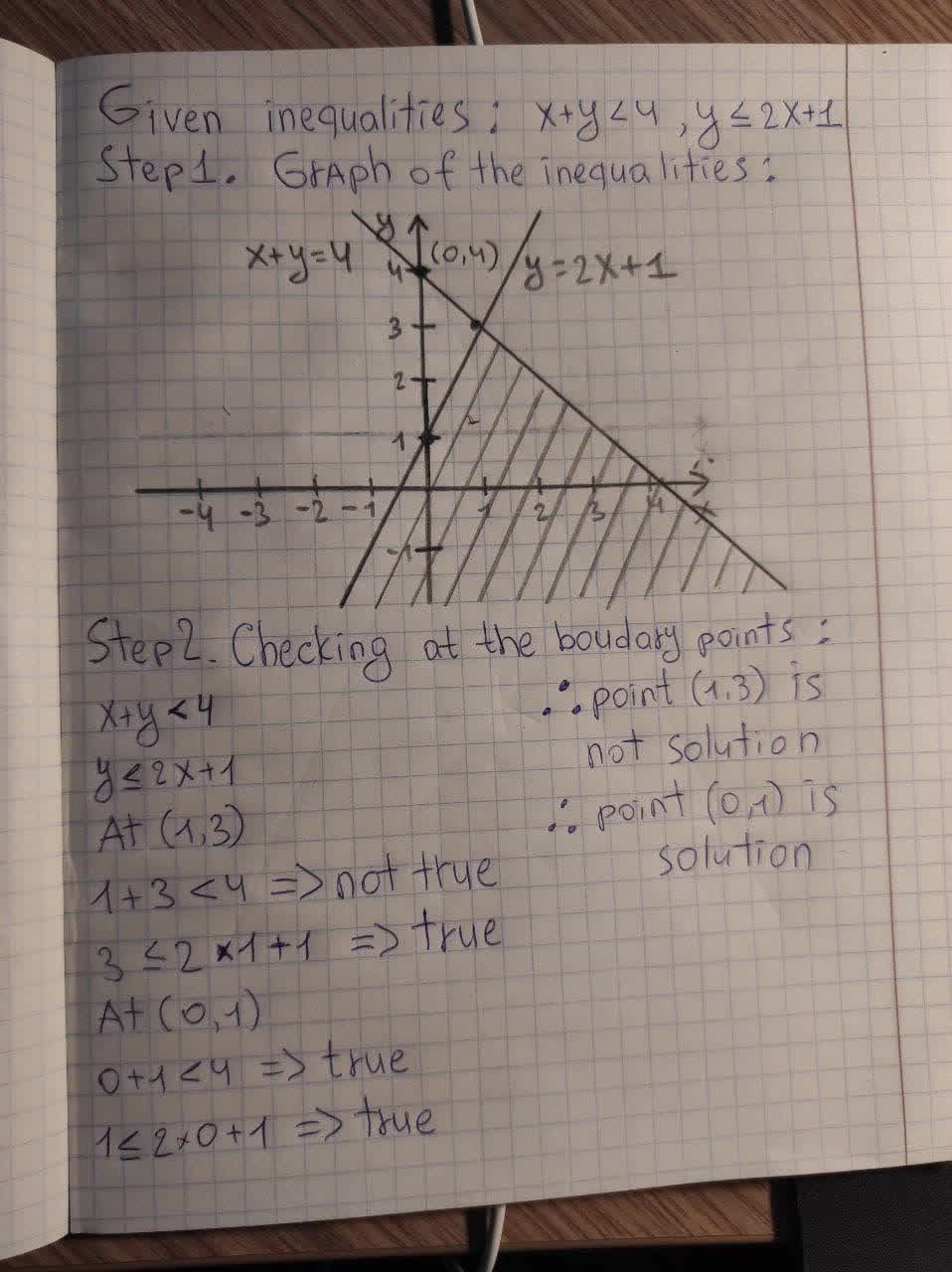# Determine whether the orderred pair is a solution to the system of inequlities x+y<4 y<=2x+1 a. (0,1) b. (3,1) c. (2,0) d. (1,4)Tabansi 2021-01-31 Answered
Determine whether the orderred pair is a solution to the system of inequlities
x+y<4
$y\le 2x+1$
a. (0,1)
b. (3,1)
c. (2,0)
d. (1,4)
You can still ask an expert for help

## Want to know more about Inequalities systems and graphs?

• Questions are typically answered in as fast as 30 minutes

Solve your problem for the price of one coffee

• Math expert for every subject
• Pay only if we can solve itunett

Step 1. Graph of the inequlitiesStep 2. Checking at the boundary points:
x+y<4
$y\le 2x+1$
At (1,3)
$1+3<4⇒$ not true
$3\le 2\cdot 1+1⇒$ true
$\therefore$point (1,3) is not solution
At(0,1)
$0+1<4⇒$ true
$1\le 2\cdot 0+1⇒$ true
$\therefore$ point (0,1) is solution